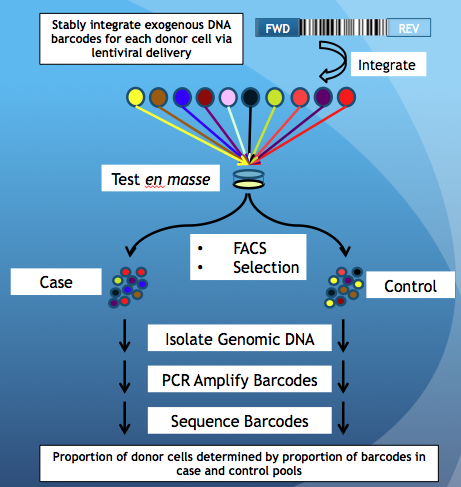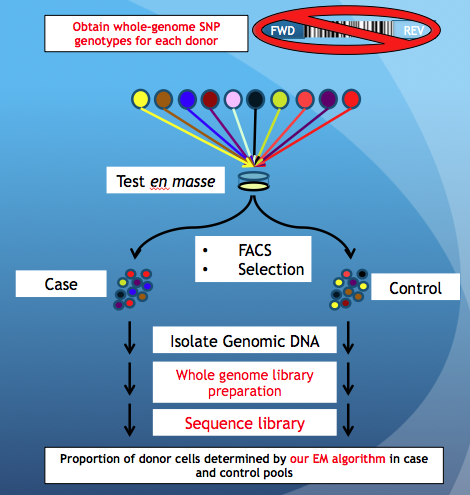## Enabling multiplexed testing of pooled donor cells through low-coverage whole genome sequencing

The software for simulating and evaluating the individual donor proportions from whole genome sequencing are available here. They are implemented in Java and therefore, Java is required for running the software. Get java here if you don't already have it.

## The Purpose of the Method

Comparing our method with the existing barcoding method.

The PRISM Barcode MethodOur MethodYu et. al., Nature Biotechnology, 2016Chan et. al., Genome Medicine, 2018

Briefly, cells are pooled together and tested using an experimental assay (FACs, Selection, etc) resulting in 2 pools of cells, being Case and Control. In PRISM, one can tell the donor proportion by amplifying and sequencing exogenous DNA barcodes that were introduced to each donor cell prior to pooling. However, our method does not require DNA barcodes, but whole-genome SNP data for each donor cell-line obtained via SNP arrays or whole-genome sequencing. The steps that are different between PRISM and our method are highlighted in red.

## Simulation

To perform simulations to determine how accurate the algorithm can predict individual donor proportion for a given scenario. The input parameters are,

• No. of individual donors.
• No. of bi-allelic autosomal SNP positions.
• Sequencing coverage per SNP.

Download the source file here and compile (javac Simulate.java). To run, perform the following on the command line.
java Simulate <No. of individuals> <No. of SNPs> <Coverage> <Outputfilename>
The parameters to the program are,
• No. of Individuals
• A positive integer, e.g. 100 or 1000 to indicate the number of unique donors within the mixed pool.
No. of SNPs A positive integer, e.g. 500000 or 1000000 to indicate the number of bi-alleleic autosomal SNPs used.
• Coverage
• A positive integer, e.g. 10 or 30 to indicate the sequencing read-depth or coverage for each SNP. The number should be less than 30000.
• Outputfilename
• The name of the outputfile that the program will write the results to. The first line of the outputfile will contain the true individual donor proportion. Subsequent lines will give the estimate of the donor proportion for each iteration of the algorithm.

## PoolSeq

To evalute the individual donor proportion on an actual dataset. The format of the dataset is as follows,
The first line is a header line and is tab-delimitted. The columns are: (1) SNP_id (2) Observed R allele count (3) Observed A allele count (4 onwards) Genotype for each individual for that SNP which can be either R/R R/A or A/A.
The subsequent lines are the actual data for the dataset. E.g.

CHR:POS:REFA:ALTA OBS_REF_A_COUNT OBS_ALT_A_COUNT PGP1:hu43860C PGP2:huC30901 PGP3:huBEDA0B PGP4:huE80E3D PGP5:hu9385BA
1:565319:G:A 90 10 R/R A/A R/R R/R R/R
1:752721:A:G 10 90 R/A A/A R/A A/A A/A
1:776546:A:G 50 50 R/A R/R A/A A/A R/R
1:777122:A:T 10 90 R/R A/A R/A A/A A/A
1:801536:T:G 85 15 R/R R/A R/A R/R R/R
1:811136:G:C 75 25 R/R R/A R/R R/R R/A
1:837657:G:C 85 15 R/A R/R R/R R/A R/R
1:841085:C:G 60 40 A/A R/A A/A R/A R/R
1:863863:G:A 80 20 R/R R/R R/R R/R R/A
1:863863:G:A 55 45 R/R R/A R/A A/A R/R

• SNP_id
• Can be any identifier that represent the SNP.
• Observed R allele count
• The number of observed reads that gives the R (Reference) allele.
• Observed A allele count
• The number of observed reads that gives the A (Alternate) allele.
• Genotype for each individual
• Can be R/R A/A or A/A representing the genotype for homozygous R (Reference), heterozygous R and A (Reference and Alternate) and homozygous A (Alternate).

Download the source file here and compile (javac PoolSeq.java). To run, perform the following on the command line,
java PoolSeq <InputfileName> <OutputfileName> [<No. of iterations>]
The InputfileName should be the name of the input file with the format as described above. The OutputfileName would be the name of the outputfile. The outputfile is a tab-delimitted file with each line being the estimate at each iteration. The final line of the file will give the final estimate of the individual donor proportion. An optional No. of iterations parameters can be used to set the number of iterations to be used. The default is 2000.

Dataset
Click here to obtain the data set used for estimating the individual proportion for PGP samples reported in the manuscript. Remember to gunzip first.